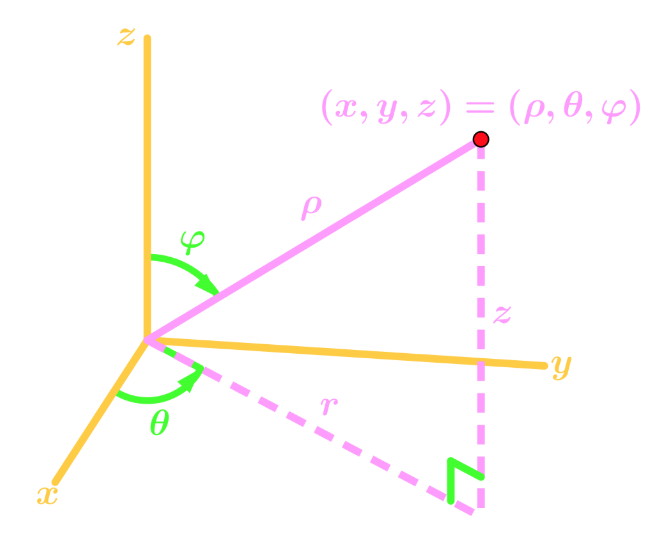# Spherical-coordinates

### Spherical-coordinates

#### Lessons

Notes:

Spherical Coordinates
Instead of Cartesian coordinates, we use spherical coordinates for situations you will see in future sections.
For now, we will just learn how to convert from 3D Cartesians coordinates to 3D spherical coordinates, and vice versa. In other words,

$(x,y,z) \to (\rho,\theta,\varphi)$

Suppose we have the following graph:From the graph, we can obtain the following equations which will be useful for converting spherical to cartesian, or vice versa:

$r = \rho \sin\varphi$
$z = \rho \cos\varphi$
$z^2 + r^2 = \rho^2$

Using the equations from last sections, we can also obtain more equations:

$x = \rho \sin\varphi \cos \theta$
$y = \rho \sin \varphi \sin \theta$
$\rho^2 = x^2 + y^2 + z^2$

• Introduction
Spherical Coordinates Overview:
a)
Spherical Coordinates
• Instead of $(x,y,z)$, we have $(\rho,\theta,\varphi)$
• Graph of the coordinates in 3D

b)
Equations to Convert from Cartesian to Spherical
• Finding the Equations
• Trig Ratios, Pythagoras
• Using Equations from last section to obtain more equations!

c)
Example of Converting Equations
• Cartesian to Spherical
• Spherical to Cartesian

• 1.
Converting Cartesian Points into Spherical Coordinates
Convert the following Cartesian Point into Spherical Coordinates:

$(2,5,1)$

• 2.
Convert the following Cartesian Point into Spherical Coordinates:

$(-2,-1,-4)$

• 3.
Converting Cartesian Equations into Spherical Coordinates
Convert the following Cartesian Equation into Spherical Coordinates:

$x^2 + y^2 - 2y = 3$

• 4.
Convert the following Cartesian Equation into Spherical Coordinates:

$\frac{x}{z} = \frac{y}{x}$

• 5.
Converting Spherical Equations into Cartesian Coordinates
Convert the following Spherical Equation into Cartesian Coordinates:

$\rho^2 = \sin\varphi \cos\theta$

• 6.
Convert the following Spherical Equation into Cartesian Coordinates

$\csc \varphi = \rho \sin \theta + \rho \cos \theta$

• 7.
Graphing the Spherical Equations by Changing into Cartesian Coordinates
Graph the following Spherical Equation in the Cartesian Plane.

$\rho^2 = 2\rho \sin\varphi \sin\theta + 4$